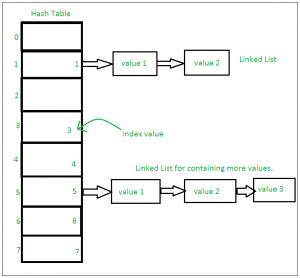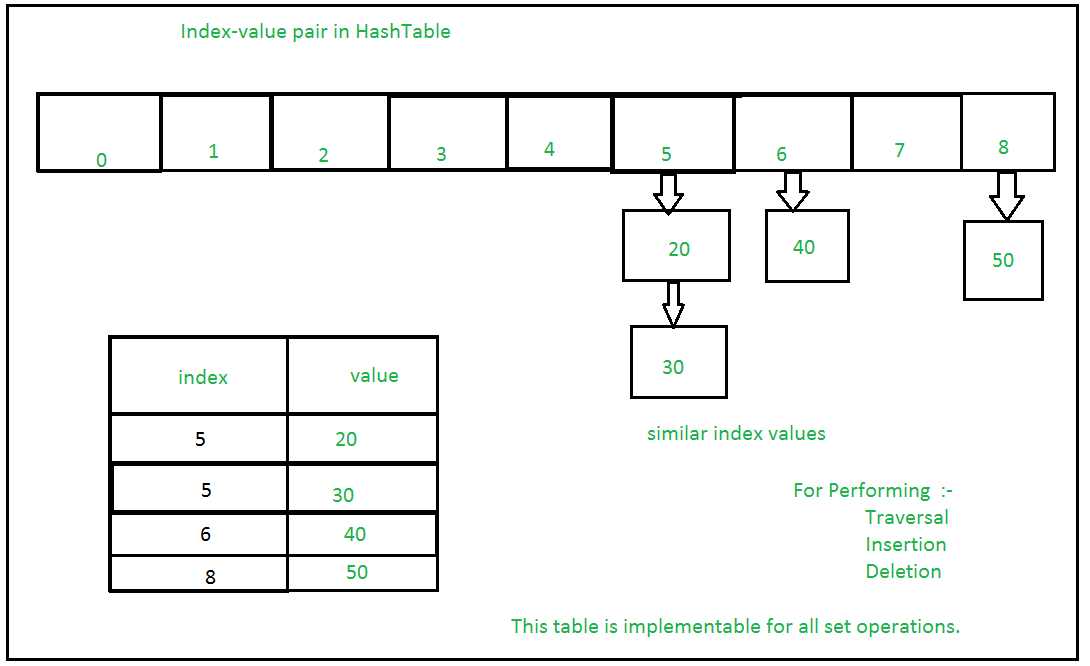# Sets in Python

A Set in Python programming is an unordered collection data type that is iterable, mutable and has no duplicate elements.

Set are represented by { } (values enclosed in curly braces)

The major advantage of using a set, as opposed to a list, is that it has a highly optimized method for checking whether a specific element is contained in the set. This is based on a data structure known as a hash table. Since sets are unordered, we cannot access items using indexes as we do in lists.

## Python3

 `var ``=` `{``"Geeks"``, ``"for"``, ``"Geeks"``}` `type``(var)`

Output:

`set`

Time Complexity: O(1)
Auxiliary Space: O(1)

### Type Casting with Python Set method

The Python set() method is used for type casting.

## Python3

 `# typecasting list to set` `myset ``=` `set``([``"a"``, ``"b"``, ``"c"``])` `print``(myset)`   `# Adding element to the set` `myset.add(``"d"``)` `print``(myset)`

Output:

Python set is an unordered datatype, which means we cannot know in which order the elements of the set are stored.

```{'c', 'b', 'a'}
{'d', 'c', 'b', 'a'}```

Time Complexity: O(n)
Auxiliary Space: O(n)

### Check unique and  Immutable with Python Set

Python sets cannot have a duplicate value and once it is created we cannot change its value.

## Python3

 `# Python program to demonstrate that` `# a set cannot have duplicate values ` `# and we cannot change its items`   `# a set cannot have duplicate values` `myset ``=` `{``"Geeks"``, ``"for"``, ``"Geeks"``}` `print``(myset)`   `# values of a set cannot be changed` `myset[``1``] ``=` `"Hello"` `print``(myset)`

Output:

The first code explains that the set cannot have a duplicate value. Every item in it is a unique value.

The second code generates an error because we cannot assign or change a value once the set is created. We can only add or delete items in the set.

```{'Geeks', 'for'}
TypeError: 'set' object does not support item assignment```

### Heterogeneous Element with Python Set

Python sets can store heterogeneous elements in it, i.e., a set can store a mixture of string, integer, boolean, etc datatypes.

## Python3

 `# Python example demonstrate that a set` `# can store heterogeneous elements` `myset ``=` `{``"Geeks"``, ``"for"``, ``10``, ``52.7``, ``True``}` `print``(myset)`

Output:

`{True, 10, 'Geeks', 52.7, 'for'}`

Time Complexity: O(n)
Auxiliary Space: O(n)

## Python Frozen Sets

Frozen sets in Python are immutable objects that only support methods and operators that produce a result without affecting the frozen set or sets to which they are applied. It can be done with frozenset() method in Python.

While elements of a set can be modified at any time, elements of the frozen set remain the same after creation.

If no parameters are passed, it returns an empty frozenset.

## Python

 `# Python program to demonstrate differences` `# between normal and frozen set`   `# Same as {"a", "b","c"}` `normal_set ``=` `set``([``"a"``, ``"b"``,``"c"``])`   `print``(``"Normal Set"``)` `print``(normal_set)`   `# A frozen set` `frozen_set ``=` `frozenset``([``"e"``, ``"f"``, ``"g"``])`   `print``(``"\nFrozen Set"``)` `print``(frozen_set)`   `# Uncommenting below line would cause error as` `# we are trying to add element to a frozen set` `# frozen_set.add("h")`

Output:

```Normal Set
{'a', 'c', 'b'}

Frozen Set
{'e', 'g', 'f'}```

Time Complexity: O(n)
Auxiliary Space: O(n)

## Internal working of Set

This is based on a data structure known as a hash table.  If Multiple values are present at the same index position, then the value is appended to that index position, to form a Linked List.

In, Python Sets are implemented using a dictionary with dummy variables, where key beings the members set with greater optimizations to the time complexity.

Set Implementation:Sets with Numerous operations on a single HashTable:## Methods for Sets

### Adding elements to Python Sets

Insertion in the set is done through the set.add() function, where an appropriate record value is created to store in the hash table. Same as checking for an item, i.e., O(1) on average. However, in worst case it can become O(n).

## Python3

 `# A Python program to` `# demonstrate adding elements` `# in a set`   `# Creating a Set` `people ``=` `{``"Jay"``, ``"Idrish"``, ``"Archi"``}`   `print``(``"People:"``, end ``=` `" "``)` `print``(people)`   `# This will add Daxit` `# in the set` `people.add(``"Daxit"``)`   `# Adding elements to the` `# set using iterator` `for` `i ``in` `range``(``1``, ``6``):` `    ``people.add(i)`   `print``(``"\nSet after adding element:"``, end ``=` `" "``)` `print``(people)`

Output:

```People: {'Idrish', 'Archi', 'Jay'}

Set after adding element: {1, 2, 3, 4, 5, 'Idrish', 'Archi', 'Jay', 'Daxit'}```

Time Complexity: O(n)
Auxiliary Space: O(n)

### Union operation on Python Sets

Two sets can be merged using union() function or | operator. Both Hash Table values are accessed and traversed with merge operation perform on them to combine the elements, at the same time duplicates are removed. The Time Complexity of this is O(len(s1) + len(s2)) where s1 and s2 are two sets whose union needs to be done.

## Python3

 `# Python Program to` `# demonstrate union of` `# two sets`   `people ``=` `{``"Jay"``, ``"Idrish"``, ``"Archil"``}` `vampires ``=` `{``"Karan"``, ``"Arjun"``}` `dracula ``=` `{``"Deepanshu"``, ``"Raju"``}`   `# Union using union()` `# function` `population ``=` `people.union(vampires)`   `print``(``"Union using union() function"``)` `print``(population)`   `# Union using "|"` `# operator` `population ``=` `people|dracula`   `print``(``"\nUnion using '|' operator"``)` `print``(population)`

Output:

```Union using union() function
{'Karan', 'Idrish', 'Jay', 'Arjun', 'Archil'}

Union using '|' operator
{'Deepanshu', 'Idrish', 'Jay', 'Raju', 'Archil'}```

Time Complexity: O(n)
Auxiliary Space: O(n)

### Intersection operation on Python Sets

This can be done through intersection() or & operator. Common Elements are selected. They are similar to iteration over the Hash lists and combining the same values on both the Table. Time Complexity of this is O(min(len(s1), len(s2)) where s1 and s2 are two sets whose union needs to be done.

## Python3

 `# Python program to` `# demonstrate intersection` `# of two sets`   `set1 ``=` `set``()` `set2 ``=` `set``()`   `for` `i ``in` `range``(``5``):` `    ``set1.add(i)`   `for` `i ``in` `range``(``3``,``9``):` `    ``set2.add(i)`   `# Intersection using` `# intersection() function` `set3 ``=` `set1.intersection(set2)`   `print``(``"Intersection using intersection() function"``)` `print``(set3)`   `# Intersection using` `# "&" operator` `set3 ``=` `set1 & set2`   `print``(``"\nIntersection using '&' operator"``)` `print``(set3)`

Output:

```Intersection using intersection() function
{3, 4}

Intersection using '&' operator
{3, 4}```

Time Complexity: O(n)
Auxiliary Space: O(n)

### Finding Differences of Sets in Python

To find differences between sets. Similar to finding differences in the linked list. This is done through difference() or – operator. Time complexity of finding difference s1 – s2 is O(len(s1))

## Python3

 `# Python program to` `# demonstrate difference` `# of two sets`   `set1 ``=` `set``()` `set2 ``=` `set``()`   `for` `i ``in` `range``(``5``):` `    ``set1.add(i)`   `for` `i ``in` `range``(``3``,``9``):` `    ``set2.add(i)`   `# Difference of two sets` `# using difference() function` `set3 ``=` `set1.difference(set2)`   `print``(``" Difference of two sets using difference() function"``)` `print``(set3)`   `# Difference of two sets` `# using '-' operator` `set3 ``=` `set1 ``-` `set2`   `print``(``"\nDifference of two sets using '-' operator"``)` `print``(set3)`

Output:

```Difference of two sets using difference() function
{0, 1, 2}

Difference of two sets using '-' operator
{0, 1, 2}```

Time Complexity: O(n)
Auxiliary Space: O(n)

### Clearing Python Sets

Set Clear() method empties the whole set inplace.

## Python3

 `# Python program to` `# demonstrate clearing` `# of set`   `set1 ``=` `{``1``,``2``,``3``,``4``,``5``,``6``}`   `print``(``"Initial set"``)` `print``(set1)`   `# This method will remove` `# all the elements of the set` `set1.clear()`   `print``(``"\nSet after using clear() function"``)` `print``(set1)`

Output:

```Initial set
{1, 2, 3, 4, 5, 6}

Set after using clear() function
set()```

Time Complexity: O(n)
Auxiliary Space: O(n)

However, there are two major pitfalls in Python sets:

1. The set doesn’t maintain elements in any particular order.
2. Only instances of immutable types can be added to a Python set.

## Operators for Sets

Sets and frozen sets support the following operators:

Recent articles on Python Set.

Whether you're preparing for your first job interview or aiming to upskill in this ever-evolving tech landscape, GeeksforGeeks Courses are your key to success. We provide top-quality content at affordable prices, all geared towards accelerating your growth in a time-bound manner. Join the millions we've already empowered, and we're here to do the same for you. Don't miss out - check it out now!

Previous
Next